Technical Test SSC JE: Electrical Engineering (EE)- 4

# Technical Test SSC JE: Electrical Engineering (EE)- 4

Test Description

## 100 Questions MCQ Test Mock Test Series for SSC JE Electrical Engineering | Technical Test SSC JE: Electrical Engineering (EE)- 4

Technical Test SSC JE: Electrical Engineering (EE)- 4 for Electrical Engineering (EE) 2022 is part of Mock Test Series for SSC JE Electrical Engineering preparation. The Technical Test SSC JE: Electrical Engineering (EE)- 4 questions and answers have been prepared according to the Electrical Engineering (EE) exam syllabus.The Technical Test SSC JE: Electrical Engineering (EE)- 4 MCQs are made for Electrical Engineering (EE) 2022 Exam. Find important definitions, questions, notes, meanings, examples, exercises, MCQs and online tests for Technical Test SSC JE: Electrical Engineering (EE)- 4 below.
Solutions of Technical Test SSC JE: Electrical Engineering (EE)- 4 questions in English are available as part of our Mock Test Series for SSC JE Electrical Engineering for Electrical Engineering (EE) & Technical Test SSC JE: Electrical Engineering (EE)- 4 solutions in Hindi for Mock Test Series for SSC JE Electrical Engineering course. Download more important topics, notes, lectures and mock test series for Electrical Engineering (EE) Exam by signing up for free. Attempt Technical Test SSC JE: Electrical Engineering (EE)- 4 | 100 questions in 100 minutes | Mock test for Electrical Engineering (EE) preparation | Free important questions MCQ to study Mock Test Series for SSC JE Electrical Engineering for Electrical Engineering (EE) Exam | Download free PDF with solutions
 1 Crore+ students have signed up on EduRev. Have you?
Technical Test SSC JE: Electrical Engineering (EE)- 4 - Question 1

### The charge on an electron is known to be 1.6 × 10–19 coulomb. In a circuit the current flowingis 1A. How many electrons will be flowing through the circuit in a second ?

Detailed Solution for Technical Test SSC JE: Electrical Engineering (EE)- 4 - Question 1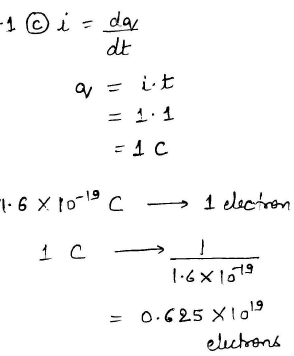Technical Test SSC JE: Electrical Engineering (EE)- 4 - Question 2

### Pyrometer is used for measurement of

Detailed Solution for Technical Test SSC JE: Electrical Engineering (EE)- 4 - Question 2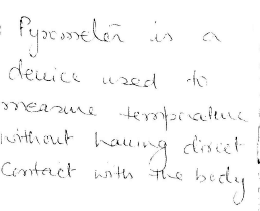Technical Test SSC JE: Electrical Engineering (EE)- 4 - Question 3

### The voltage and current waveforms for an elements are shown in the figures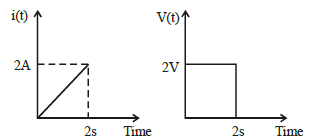The circuit element and its value are

Detailed Solution for Technical Test SSC JE: Electrical Engineering (EE)- 4 - Question 3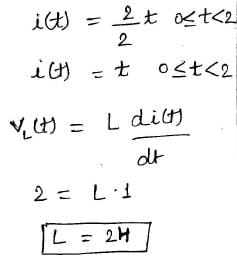Technical Test SSC JE: Electrical Engineering (EE)- 4 - Question 4

A magnetic ring has a mean circumference of 20 cm and a cross section of 20 cm2 and has 800 numbers of turns of wire. When the exciting current is 5A, the flux is 2 mWb. The relative permeability of iron is nearly

Detailed Solution for Technical Test SSC JE: Electrical Engineering (EE)- 4 - Question 4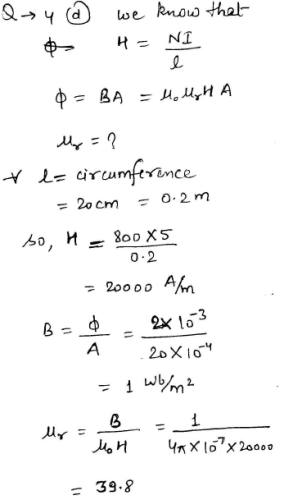Technical Test SSC JE: Electrical Engineering (EE)- 4 - Question 5

Random error is

Detailed Solution for Technical Test SSC JE: Electrical Engineering (EE)- 4 - Question 5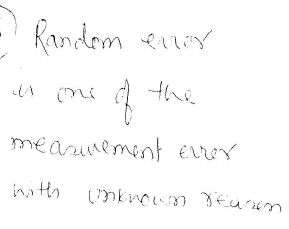Technical Test SSC JE: Electrical Engineering (EE)- 4 - Question 6

A semiconductor device made out of a material having very high temperature coefficient of resistance is

Technical Test SSC JE: Electrical Engineering (EE)- 4 - Question 7

One single-phase energy meter operating on 230 V and 5A for 5 hours makes 1940 revolution. Meter constant is 400 rev/kWh. The power factor of the load is

Detailed Solution for Technical Test SSC JE: Electrical Engineering (EE)- 4 - Question 7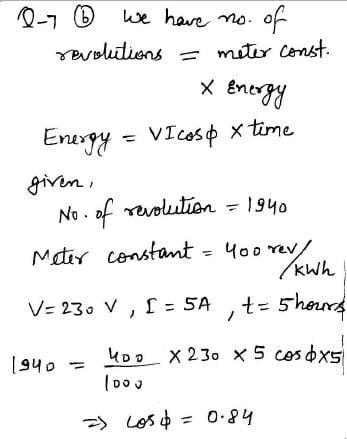Technical Test SSC JE: Electrical Engineering (EE)- 4 - Question 8

In a junction transistor, the collector cut-off current 'ICBO' reduces considerably by doping the

Detailed Solution for Technical Test SSC JE: Electrical Engineering (EE)- 4 - Question 8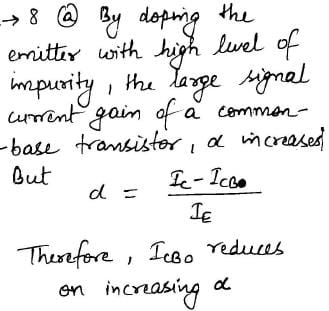Technical Test SSC JE: Electrical Engineering (EE)- 4 - Question 9

In a D.C. generator all of the following could be the effects of iron losses except

Technical Test SSC JE: Electrical Engineering (EE)- 4 - Question 10

The losses occurring in a D.C. generator are given below which loss is likely to have highest proportion at rated load of the generator

Technical Test SSC JE: Electrical Engineering (EE)- 4 - Question 11

In a D.C. generator the critical resistance can be increased by

Technical Test SSC JE: Electrical Engineering (EE)- 4 - Question 12

A series resonant circuit has an inductive reactance of 1000W, a capacitive reactance of 1000W and a resistance of 0.1W. If resonant frequency is 10 MHz, then the bandwidth of the circuit will be

Detailed Solution for Technical Test SSC JE: Electrical Engineering (EE)- 4 - Question 12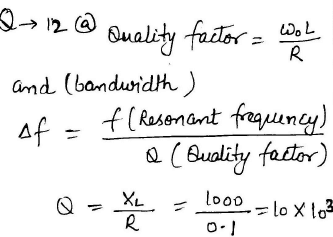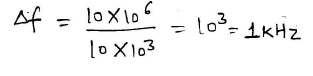Technical Test SSC JE: Electrical Engineering (EE)- 4 - Question 13

Consider the network given below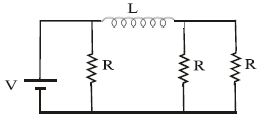The time constant of the circuit is

Detailed Solution for Technical Test SSC JE: Electrical Engineering (EE)- 4 - Question 13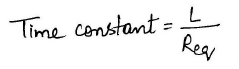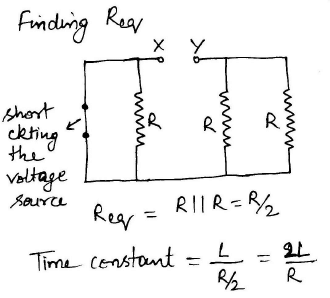Technical Test SSC JE: Electrical Engineering (EE)- 4 - Question 14

Two bulbs marked 200 watt, 250 volts and 100 watt -250 volts are joined in series to 250 volts supply. Power consumed in circuits is

Detailed Solution for Technical Test SSC JE: Electrical Engineering (EE)- 4 - Question 14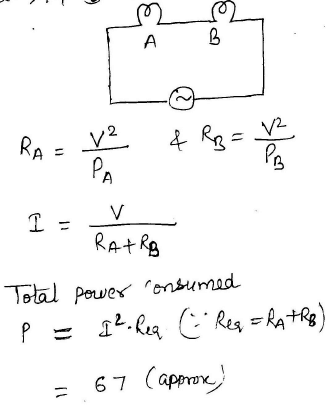Technical Test SSC JE: Electrical Engineering (EE)- 4 - Question 15

Three equal resistors connected in series across a source of emf together dissipate 10 watts of power. What would be the power dissipated in the same resistors when they are connected in parallel across the same source of emf ?

Detailed Solution for Technical Test SSC JE: Electrical Engineering (EE)- 4 - Question 15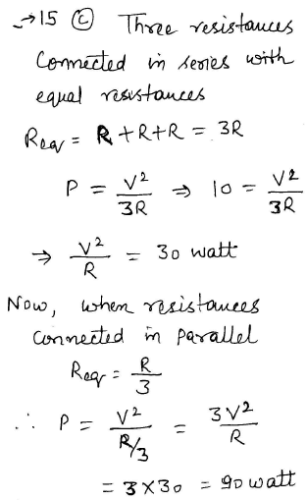Technical Test SSC JE: Electrical Engineering (EE)- 4 - Question 16

In a transmission system, the weight of copper used is proportional to

Technical Test SSC JE: Electrical Engineering (EE)- 4 - Question 17

If the frequency of a transmission system is changed from 50 Hz to 100 Hz, the string efficiency will

Technical Test SSC JE: Electrical Engineering (EE)- 4 - Question 18

A piece of silver wire has a resistance of 1W. A manganin wire has specific resistance 30 times that of silver. The resistance of a manganin wire of one fourth length and one third diameter will be

Detailed Solution for Technical Test SSC JE: Electrical Engineering (EE)- 4 - Question 18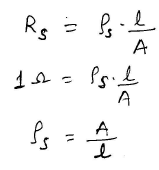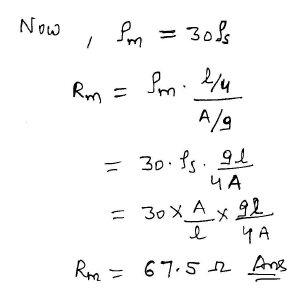Technical Test SSC JE: Electrical Engineering (EE)- 4 - Question 19

Wattless current is possible, only in

Technical Test SSC JE: Electrical Engineering (EE)- 4 - Question 20

If the stator voltage and frequency of an induction motor are reduced proportionately,its

Technical Test SSC JE: Electrical Engineering (EE)- 4 - Question 21

The efficiency and p.f. of SCIM increases in proportion to its

Technical Test SSC JE: Electrical Engineering (EE)- 4 - Question 22

Which of the following lamp gives nearly monochromatic light ?

Technical Test SSC JE: Electrical Engineering (EE)- 4 - Question 23

A auto transformer used with sodium vapour lamp should have

Technical Test SSC JE: Electrical Engineering (EE)- 4 - Question 24

For supply on 25 kV, 50 Hz single phase, suitable motor for electric traction is

Technical Test SSC JE: Electrical Engineering (EE)- 4 - Question 25

The welding transformer used in resistance welding will

Technical Test SSC JE: Electrical Engineering (EE)- 4 - Question 26

The power factor of the load using welding transformer is usually

Technical Test SSC JE: Electrical Engineering (EE)- 4 - Question 27

Technical Test SSC JE: Electrical Engineering (EE)- 4 - Question 28

Pump, blowers and fans are usually called for

Technical Test SSC JE: Electrical Engineering (EE)- 4 - Question 29

In lap windings, the equalizer rings are used to save

Technical Test SSC JE: Electrical Engineering (EE)- 4 - Question 30

A transformer is considered to have a network of series capacitance due to

Technical Test SSC JE: Electrical Engineering (EE)- 4 - Question 31

Stator voltage control for speed control of induction motors is suitable for

Technical Test SSC JE: Electrical Engineering (EE)- 4 - Question 32

The equivalent inductance for the following inductive circuit is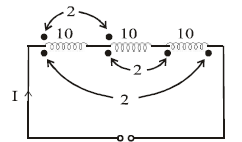Detailed Solution for Technical Test SSC JE: Electrical Engineering (EE)- 4 - Question 32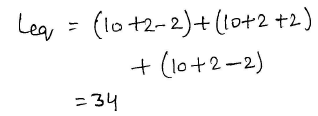Technical Test SSC JE: Electrical Engineering (EE)- 4 - Question 33

A particular current is made up of two components a 10A dc and a sinusoidal current of peak value of 14.14A. The average value of resultant current is

Technical Test SSC JE: Electrical Engineering (EE)- 4 - Question 34

The unit of a thermal resistance of a semiconductor device is

Technical Test SSC JE: Electrical Engineering (EE)- 4 - Question 35

Assertion (A) : FETs are more suitable at the input stages of millivoltmeter and CROs than
BJTs
Reason (R) : A FET has lower output impedance than a BJT

Technical Test SSC JE: Electrical Engineering (EE)- 4 - Question 36

With 100% inductive shunt compensation, the voltage profile is flat for

Technical Test SSC JE: Electrical Engineering (EE)- 4 - Question 37

Three phase transformers which cannot be connected in parallel are

Technical Test SSC JE: Electrical Engineering (EE)- 4 - Question 38

In case of a 3-phase short circuit in a system, the power fed into the system is

Technical Test SSC JE: Electrical Engineering (EE)- 4 - Question 39

The most economical limit of power factor correcting is governed by

Detailed Solution for Technical Test SSC JE: Electrical Engineering (EE)- 4 - Question 39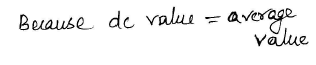Technical Test SSC JE: Electrical Engineering (EE)- 4 - Question 40

As per I.E. rules the insulation resistance between conductor and earth should not be less than

Detailed Solution for Technical Test SSC JE: Electrical Engineering (EE)- 4 - Question 40

As per IE rules, insulation Resistance between earth and conductor should not exceed the 1/5000 time of the current flowing through the conductor.

That is to be measured by the ‘Megger’ instrument.

As per IE rules, 1956 insulation Resistance between earth and conductor should in range of mega Ohms.

IMPORTANT TO REMEMBER:-

One Mega ohm Rule for IR Value for Equipment

Based upon equipment rating:

< 1K V = 1 MΩ minimum
>1KV = 1 MΩ /1KV

Therefore, based on this analogy for Medium and High voltages there is need for Insulation –

Therefore,

Value of insulation Resistance between earth and conductor should not be less than 100 M ohm.

Technical Test SSC JE: Electrical Engineering (EE)- 4 - Question 41

Excess carriers are generated in a sample of N-type semiconductor by shining light at one end. The current flow in the sample will be made up of

Technical Test SSC JE: Electrical Engineering (EE)- 4 - Question 42

The effective resistance between the terminals A and B in the circuit shown in the figure is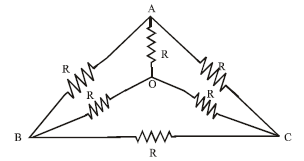Detailed Solution for Technical Test SSC JE: Electrical Engineering (EE)- 4 - Question 42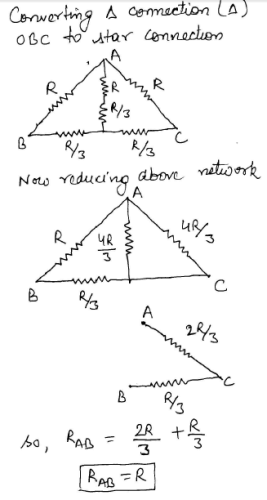Technical Test SSC JE: Electrical Engineering (EE)- 4 - Question 43

In the circuit of figure below, the voltage V(t) is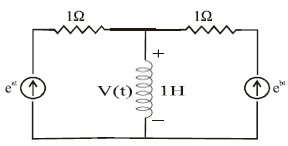Detailed Solution for Technical Test SSC JE: Electrical Engineering (EE)- 4 - Question 43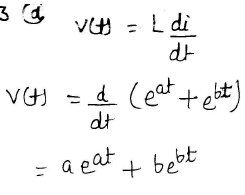Technical Test SSC JE: Electrical Engineering (EE)- 4 - Question 44

A dc shunt generator builds up to a voltage of 220 V at no load while running at its rated speed. If the speed of the generator is raised by 25% keeping the circuit conditions unaltered,then the voltage to which the machine would build up will

Detailed Solution for Technical Test SSC JE: Electrical Engineering (EE)- 4 - Question 44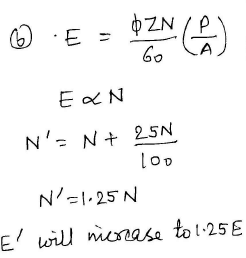Technical Test SSC JE: Electrical Engineering (EE)- 4 - Question 45

In normal dc machines operating at full load conditions the most powerful electromagnet is

Detailed Solution for Technical Test SSC JE: Electrical Engineering (EE)- 4 - Question 45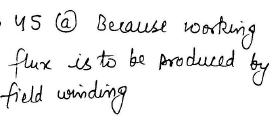Technical Test SSC JE: Electrical Engineering (EE)- 4 - Question 46

If the secondary winding of the ideal transformer shown in the circuit of figure has 40 turns, the number of turns in the primary winding for maximum power transfer to the 2 resistor will be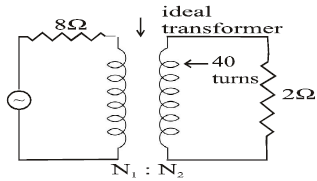Detailed Solution for Technical Test SSC JE: Electrical Engineering (EE)- 4 - Question 46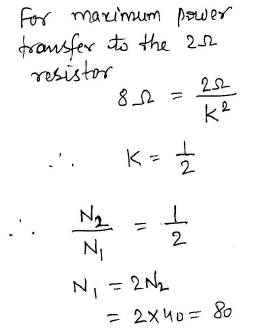Technical Test SSC JE: Electrical Engineering (EE)- 4 - Question 47

In the circuit shown in the figure, if the ammeter indicates 1A and the voltmeter having an internal resistance of 1KW indicated 100V, then the value of R would be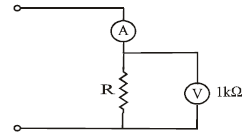Detailed Solution for Technical Test SSC JE: Electrical Engineering (EE)- 4 - Question 47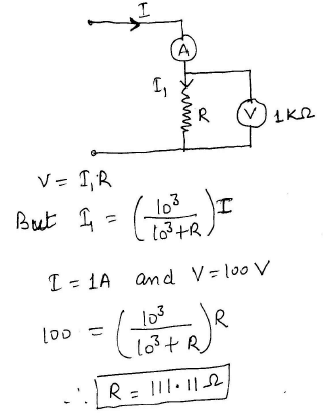Technical Test SSC JE: Electrical Engineering (EE)- 4 - Question 48

A Galvanometer has

Technical Test SSC JE: Electrical Engineering (EE)- 4 - Question 49

Thermal runaway is not possible in FET because as the temperature of FET increases

Technical Test SSC JE: Electrical Engineering (EE)- 4 - Question 50

Which of the following statement is correct ?

Technical Test SSC JE: Electrical Engineering (EE)- 4 - Question 51

In an actual transformer the iron loss remains practically constant from no load to full load because

Technical Test SSC JE: Electrical Engineering (EE)- 4 - Question 52

A 2 pole generator with wave wound armature has 51 slots each having 24 conductors. The flux per pole is 0.01 Wb. The speed at which the generator should be run to induce the voltage of 220 should be

Detailed Solution for Technical Test SSC JE: Electrical Engineering (EE)- 4 - Question 52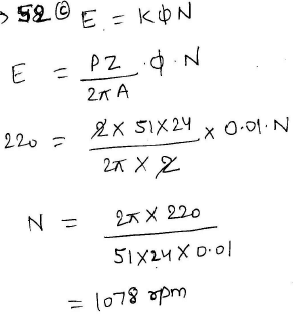Technical Test SSC JE: Electrical Engineering (EE)- 4 - Question 53

For parallel operation, the generators normally preferred are

Technical Test SSC JE: Electrical Engineering (EE)- 4 - Question 54

Which winding on dc generators is preferred for generating large currents ?

Technical Test SSC JE: Electrical Engineering (EE)- 4 - Question 55

The advantage of high voltage transmission is are

Technical Test SSC JE: Electrical Engineering (EE)- 4 - Question 56

The function of steel wire in a ACSR conductor is

Technical Test SSC JE: Electrical Engineering (EE)- 4 - Question 57

The flicker effect of fluorescent lamp is more pronounced at

Technical Test SSC JE: Electrical Engineering (EE)- 4 - Question 58

The level of illumination on a surface least depends on

Technical Test SSC JE: Electrical Engineering (EE)- 4 - Question 59

In resistance welding, aluminium as compared to steel, requires

Technical Test SSC JE: Electrical Engineering (EE)- 4 - Question 60

Pole changing method of speed control is used in

Technical Test SSC JE: Electrical Engineering (EE)- 4 - Question 61

The resistance of series RLC circuit is doubled and inductance is halved, then BW becomes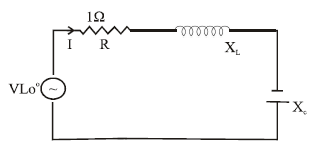Technical Test SSC JE: Electrical Engineering (EE)- 4 - Question 62

The input impedance of CRO is nearly

Technical Test SSC JE: Electrical Engineering (EE)- 4 - Question 63

Manometer is used for measurement of

Technical Test SSC JE: Electrical Engineering (EE)- 4 - Question 64

Centrifugal pumps are usually driven by

Technical Test SSC JE: Electrical Engineering (EE)- 4 - Question 65

A 10 mF capacitor was tested by a multimeter using resistance measuring range. If the multimeter reads zero, it can be concluded that

Technical Test SSC JE: Electrical Engineering (EE)- 4 - Question 66

Which of the following induction motors will have the least shaft diameter ?

Technical Test SSC JE: Electrical Engineering (EE)- 4 - Question 67

A 20 kW, 4 pole, 400 V, 60 Hz, 3 phase, 1728 rpm induction motor is connected to 400V, 50 Hz supply, the motor will

Technical Test SSC JE: Electrical Engineering (EE)- 4 - Question 68

In a circuit breaker the active recovery voltage depends upon

Technical Test SSC JE: Electrical Engineering (EE)- 4 - Question 69

Which of the following technique/method is used for the measurement of ac high frequency voltages ?

Technical Test SSC JE: Electrical Engineering (EE)- 4 - Question 70

Boosters are basically

Technical Test SSC JE: Electrical Engineering (EE)- 4 - Question 71

Which of the following short circuit is the most dangerous ?

Technical Test SSC JE: Electrical Engineering (EE)- 4 - Question 72

A capacitor start, capacitor run single phase induction motor is basically a

Technical Test SSC JE: Electrical Engineering (EE)- 4 - Question 73

In squirrel cage induction motor, the starting current is

Technical Test SSC JE: Electrical Engineering (EE)- 4 - Question 74

The input gate current of FET is closer to

Technical Test SSC JE: Electrical Engineering (EE)- 4 - Question 75

The magnetising force on an iron ring is 1750 AT/m when the flux density is 1.1 T. The relative permeability of iron is

Detailed Solution for Technical Test SSC JE: Electrical Engineering (EE)- 4 - Question 75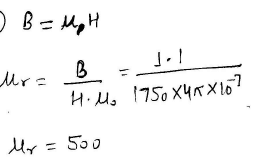Technical Test SSC JE: Electrical Engineering (EE)- 4 - Question 76

Millman's theorem yields

Technical Test SSC JE: Electrical Engineering (EE)- 4 - Question 77

A 10 mH inductor carries a sinusoidal current of 1A rms at a frequency of 50 Hz. The average power dissipated by the inductor is

Detailed Solution for Technical Test SSC JE: Electrical Engineering (EE)- 4 - Question 77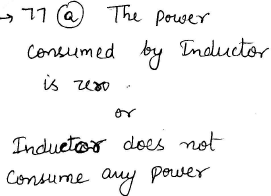Technical Test SSC JE: Electrical Engineering (EE)- 4 - Question 78

Silicon diode is less suited for low voltage rectifier operation because

Technical Test SSC JE: Electrical Engineering (EE)- 4 - Question 79

The resistivity of a semi-conductor

Detailed Solution for Technical Test SSC JE: Electrical Engineering (EE)- 4 - Question 79

Resistivity of conductors increases with increase in temperature because rate of collisions between free electrons and ions increase with increase of temperature. However, the resistivity of semiconductors decreases with increase in temperature, because more and more covalent bonds are broken at higher temperatures.

Technical Test SSC JE: Electrical Engineering (EE)- 4 - Question 80

In case of induction motor, with increase in supply voltage, which of the following increases?

Detailed Solution for Technical Test SSC JE: Electrical Engineering (EE)- 4 - Question 80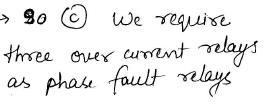Technical Test SSC JE: Electrical Engineering (EE)- 4 - Question 81

In case of transformers regulation varies as

Technical Test SSC JE: Electrical Engineering (EE)- 4 - Question 82

In a transformer, with change in frequency

Technical Test SSC JE: Electrical Engineering (EE)- 4 - Question 83

Sometimes a reactor is connected in series with a transformer to

Technical Test SSC JE: Electrical Engineering (EE)- 4 - Question 84

Damping torque in instruments is generally not produced

Technical Test SSC JE: Electrical Engineering (EE)- 4 - Question 85

In hot wire instruments, the sensing wire is made of

Technical Test SSC JE: Electrical Engineering (EE)- 4 - Question 86

When a multiplier is added to an existing voltmeter for extending its range, its electromagnetic damping

Technical Test SSC JE: Electrical Engineering (EE)- 4 - Question 87

In an insulated cable having core diameter d and overall diameter D, the ratio of maximum
to minimum dielectric stress is given by

Technical Test SSC JE: Electrical Engineering (EE)- 4 - Question 88

When the damping force is more than the optimum, the instrument will become

Technical Test SSC JE: Electrical Engineering (EE)- 4 - Question 89

In a moving iron type ammeter, the coil has

Technical Test SSC JE: Electrical Engineering (EE)- 4 - Question 90

How many relays are used to detect inter phase fault of a three line system ?

Technical Test SSC JE: Electrical Engineering (EE)- 4 - Question 91

If δ is the loss angle of the cable, its power factor is

Detailed Solution for Technical Test SSC JE: Electrical Engineering (EE)- 4 - Question 91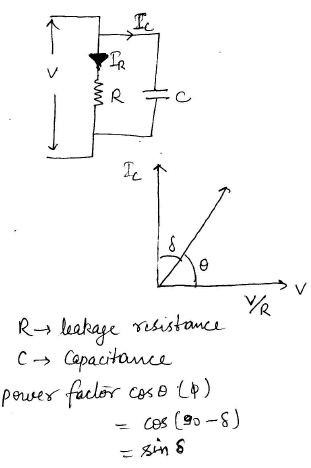Technical Test SSC JE: Electrical Engineering (EE)- 4 - Question 92

The critical clearing time of a fault in power system is related to

Technical Test SSC JE: Electrical Engineering (EE)- 4 - Question 93

The depletion layer across a p+–n junction lies

Technical Test SSC JE: Electrical Engineering (EE)- 4 - Question 94

A combination of two diodes connected in parallel when compared to a single diode can with stand

Technical Test SSC JE: Electrical Engineering (EE)- 4 - Question 95

Megger is a combination of

Technical Test SSC JE: Electrical Engineering (EE)- 4 - Question 96

In a separately excited generator, field winding is excited by

Technical Test SSC JE: Electrical Engineering (EE)- 4 - Question 97

In an RLC parallel circuit, the impedance at resonance is

Detailed Solution for Technical Test SSC JE: Electrical Engineering (EE)- 4 - Question 97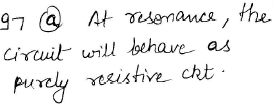Technical Test SSC JE: Electrical Engineering (EE)- 4 - Question 98

Which one of the following theorem is a manifestation of the law of conservation of energy ?

Technical Test SSC JE: Electrical Engineering (EE)- 4 - Question 99

Resistivity of earth increases sharply if the moisture falls below

Technical Test SSC JE: Electrical Engineering (EE)- 4 - Question 100

Which type of drive can be used for hoisting machinery

## Mock Test Series for SSC JE Electrical Engineering

2 videos|1 docs|55 tests
 Use Code STAYHOME200 and get INR 200 additional OFF Use Coupon Code
Information about Technical Test SSC JE: Electrical Engineering (EE)- 4 Page
In this test you can find the Exam questions for Technical Test SSC JE: Electrical Engineering (EE)- 4 solved & explained in the simplest way possible. Besides giving Questions and answers for Technical Test SSC JE: Electrical Engineering (EE)- 4, EduRev gives you an ample number of Online tests for practice

## Mock Test Series for SSC JE Electrical Engineering

2 videos|1 docs|55 tests